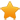Switch Editions?
CancelChannel: CodeSection,代码区,网络安全 - CodeSec
Viewing all articles

# Were there more notable deaths than expected in 2016?

0
0

After exploring mystudy population of Wikipedia deaths, I want to analyse the time series of monthly counts of notable deaths. This is not a random interest of mine, my PhD thesis was about monitoring time series of count, the application being weekly number of reported cases of various diseases.

Number of deaths reported over time library("ggplot2") library("viridis") library("dplyr") library("lubridate") deaths <- readr::read_csv("data/deaths_with_demonyms.csv") deaths %>% group_by(date) %>% summarize(n_deaths = n()) %>% ggplot(aes(x = date, xend = date, y = 0, yend = n_deaths)) + geom_segment() + xlab("Time (days)") + ylab("Number of reported deaths")

There is an increase in the number of reported deaths over time, which is not surprising given the growth of Wikipedia.

There are three major peaks. They are due to the 2010 Haiti earthquake of the 12th of January; the 2010 Polish Air Force Tu-154 crash on the 10th of April of 2010; and the 2011 Lokomotiv Yaroslavl air disaster on the 7th of September 2011. In the following, I will remove the fatalities from these events.

Modeling the time series

When I think of modeling time series of count in R, I either think of surveillance , which was the implementational repository of my methodological developments as Michael would say ; or of tscount , which was the package of my PhD writing buddy Tobias Liboschik (after meeting at a conference we exchanged feedback on our thesis chapters which was awesome). If you have an interest in prospectively detecting outbreaks and a time series with seasonality, like the time series of number of cases of Salmonella, then surveillance is the best choice. Now in the case of Wikipedia deaths, I felt more inclined towards using tscount . I had never properly used it apart from a comparison section in my thesis so it was also more exciting. So tscount it was!

In tscount , you have tsglm models which take into account both the count nature of the data and its time series nature, i.e. you can treat auto-correlation. The vignette of tscount is a really great manuscript.

I’ll use the time series of monthly counts of deaths in the rest of the post.

library("tscount") monthly_deaths <- deaths %>% group_by(wiki_link) %>% mutate(month = update(date, day = 1)) %>% ungroup() %>% group_by(month) %>% summarize(n = n()) haiti_earthquake <- update(ymd("2010-01-12"), day = 1) aircrash1 <- update(ymd("2010-01-12"), day = 1) aircrash2 <- update(ymd("2011-09-07"), day = 1) monthly_deaths <- mutate(monthly_deaths, n = ifelse(month == haiti_earthquake, n - 10, n)) monthly_deaths <- mutate(monthly_deaths, n = ifelse(month == aircrash1, n - 96, n)) monthly_deaths <- mutate(monthly_deaths, n = ifelse(month == aircrash2, n - 44, n)) ggplot(monthly_deaths) + geom_segment(aes(x = month, xend = month, y = 0, yend = n)) + ylab("Number of reported deaths") + xlab("Time (months)")

We still see the upward trend, and I have a feeling we’re also dealing with overdispersion. First step before doing any modeling: getting a ts object. I hold off the last 12 months which are 2016 months.

ts_deaths <- xts::xts(monthly_deaths\$n[1:144], monthly_deaths\$month[1:144]) ts_deaths = ts(ts_deaths, freq=12, start=c(2004, 1)) plot(ts_deaths)

Then I use a model with regression on the past observation, on the mean from one year ago, and with a temporal trend. I log-transform time because the regression model uses a log link and I don’t expect the time trend to be exponential on the response scale. I’ll fit a Poisson and a negative binomial models in order to compare them.

time <- log(1:nrow(monthly_deaths)) fit_pois <- tsglm(ts_deaths[1:144], model = list(past_obs = 1, past_mean = 13), xreg = time[1:144], distr = "poisson") fit_nb <- tsglm(ts_deaths[1:144], model = list(past_obs = 1, past_mean = 13), xreg = time[1:144], distr = "nbinom")

For choosing between the two models I’ll use scoring rules.

rbind(Poisson = scoring(fit_pois), NegBin = scoring(fit_nb)) ## logarithmic quadratic spherical rankprob dawseb normsq ## Poisson 10.23428 0.003338134 -0.0495852 40.83497 17.633417 11.8105665 ## NegBin 5.68638 -0.004157600 -0.0655695 35.61633 9.417219 0.9722222 ## sqerror ## Poisson 3835.296 ## NegBin 3835.296

The smaller the scoring rule the better, so we should use the negative binomial model which I’m happy about since I had assumed we’d be dealing with overdispersion. Note that I used scoring rules whose values were computed on the part of the data I used for fitting the model, which is what Tobias Liboschik did in his manuscript for the Campylobacter example.

Predicting 2016 values

I realized there’s no broom “adapter” for tsglm so I’ll write untidy code and hope you’ll all forgive me.

set.seed(1) pred2016 <- predict(fit_nb, n.ahead = 12, level = 0.9, global = TRUE, B = 3000, newxreg = time[145:156]) monthly_deaths <- mutate(monthly_deaths, lower = NA, upper = NA, pred = NA) monthly_deaths\$lower[145:156] <- pred2016\$interval[,1] monthly_deaths\$upper[145:156] <- pred2016\$interval[,2] monthly_deaths\$pred[145:156] <- pred2016\$pred

Let’s plot the result.

ggplot(monthly_deaths) + geom_segment(aes(x = month, xend = month, y = 0, yend = n)) + ylab("Number of reported deaths") + xlab("Time (months)") + geom_line(aes(x = month, y = pred), col = "blue") + geom_ribbon(aes(x = month, ymin = lower, ymax = upper), alpha = 0.5)

So what? All number of deaths of 2016 are in the 90% coverage prediction interval computed by tscount which means that maybe 2016 wasn’t a bad year for notable deaths in general , but then one should make the same analysis on a subset of the data like notable dead from the arts by filtering them according to their role, or notable dead whose Wikipedia pages consistently had more than X% of total Wikipedia daily page views before their deaths. I’m actually planning on doing more with page views, but in the meantime, it was nice to play with time series of counts again!

I’d like to end this post with a note from my husband, who thinks having a blog makes me an influencer. If you too like Wikipedia, consider donating to the Wikimedia foundation .

Viewing all articles

More Pages to Explore .....